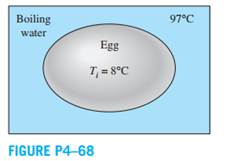Create an Account

Home / Questions / An ordinary egg can be approximated as a 55 cmdiameter sphere whose properties are roughly

An ordinary egg can be approximated as a 55 cmdiameter sphere whose properties are roughly

An ordinary egg can be approximated as a 5.5-cmdiameter sphere whose properties are roughly k = 0.6 W/m·K and α = 0.14 × 10-6 m2/s. The egg is initially at a uniform temperature of 8°C and is dropped into boiling water at 97°C. Taking the convection heat transfer coefficient to be h = 1400 W/m2·K, determine how long it will take for the center of the egg to reach 70°C. Solve this problem using analytical one-term approximation method (not the Heisler charts).Jul 24 2020 View more View LessSubscribe To Get Solution